# KaTeX - a new way to display math on the Web

By Murray Bourne, 17 Sep 2014

UPDATE (Jul 2015): KaTeX has undergone various improvements over the last few months. It is now capable of handling more environments than before (e.g. it can now do matrices.) The demo page mentioned below indicates the latest.

Ben Alpert and Emily Eisenberg at Khan Academy have just released a promising new way to deliver math on the Web, called KaTeX.

It's a direct competitor to MathJax, which I've been using on IntMath for some time now.

## KaTeX is certainly faster

You can see immediately that KaTeX produces math much faster than MathJax. This is because KaTex "renders its math synchronously and doesn't need to reflow the page", according to their short blurb on Github.

There is obviously much less time required for processing. KaTeX doesn't suffer from the page reflow jumps that MathJax has after each equation is created. (To be fair, there are ways around those instabilities in MathJax.)

I wrote a page so you can see the same input as processed by both methods. See

There's a rough "time to process page" at the top. It's not a fair comparison in the sense that KaTeX doesn't handle several of the equations (yet), and MathJax handles them all.

The difference is stark on a mobile phone - around 1 second for KaTeX and around 30 seconds for MathJax.

## KaTeX works in modern browsers

It claims to "supports all major browsers, including Chrome, Safari, Firefox, Opera, and IE 8 - IE 11". So does MathJax, and it also copes with the vagaries of earlier IE versions.

So far the only differences I've noted are some font weight issues. In Chrome, the rendering seems "thin" to me. Sometimes it's too much so, and the "equal" signs almost look like minuses, and some minus signs almost disappear.

But that is not such an issue in Firefox or IE. Here are some screen shot comparisons.

Using KaTeX in Chrome, where "=" and "−" are thin :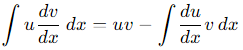Using MathJax in Chrome is bolder, and easier to read: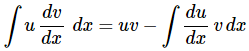Here's how KaTeX looks in Firefox (somewhere between the first 2, and most pleasing to me):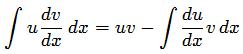KaTeX in Internet Explorer looks almost identical to the above FF rendering, but has some alignment issues. (Note where the u and v are in relation to the fraction line.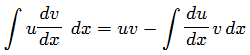## IE has problems, as usual

It's no surprise that IE falls over here and there. It almost always does. Here are 2 instances that I've spotted so far: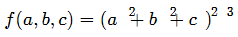The right hand side should have been: (a2 + b2 + c2)3

This surd also has obvious problems in IE: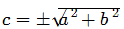## KaTeX is a work in progress

As mentioned earlier, KaTeX doesn't do everything yet, but it's under constant development. You can see what currently works on the Demo page.

This list on the GitHub KaTeX wiki shows what functions are currently available.

## How to use KaTeX in your page

The setup for KaTeX is similar to what you have to do with MathJax, but there is no CDN version of KaTeX yet.

Extract the zip amd upload it all to your server. (This is much smaller than MathJax, as there is no image fallback with KaTeX, so there aren't thousands of images to upload.)

Next, in the head of your page, point towards the KaTeX javascript and css, something like this:

<link rel="stylesheet" type="text/css"
href="/path/to/katex/katex.min.css">
<script type="text/javascript"
src="/path/to/katex/katex.min.js"></script>

If you have only a few equations on your page, you can proceed as follows:

<p><span id="mykatex1">...</span></p><script>
katex.render("f(a,b,c) = (a^2+b^2+c^2)^3", mykatex1);
</script> 

This will place the equation into your Web page, as properly rendered math.

A second equation would need a new id on the span, and in the script, like this:

<p><span id="mykatex2">...</span></p><script>
katex.render("f(a,b,c) = (a^2+b^2+c^2)^3", mykatex2);
</script> 

### If you have many equations on your page

If you want to present a lot of LaTeX on your page, it would be better to proceed as follows.

You would use the same class name for the DIVs (or spans) containing math. Your HTML would look something like:

<div class="math">
f(x) = \sqrt{1+x} \quad (x \ge  -1)  </div><p>Some other text here. </p><div class="math">
f(x) = \sqrt{1+x}, \quad x \ge -1  </div>


Next, we use javascript to iterate over all the DIVs with class "math" (they can be <p>, <span> or <td> too, as long as the class is "math" and it contains LaTeX only.

I'm using jQuery to do this, but there are pure javascript methods as well.

The following code just means:

1. Iterate over all the objects with class name "math"
2. Get the text from the object (this is the LaTeX that we want to convert)
3. Get the type of element. If it's a DIV, then add "\displaystyle" so it will be presented as math centered on the page.
4. Try to convert it to math using katex.render.
5. If it fails, output an error message.
(function(){
$(".math").each(function() { var texTxt =$(this).text();
el = $(this).get(0); if(el.tagName == "DIV"){ addDisp = "\\displaystyle"; } else { addDisp = ""; } try { katex.render(addDisp+texTxt, el); } catch(err) {$(this).html("<span class='err'>"+err);
}
});
})();


## Generating HTML on the server

There's also an option to generate HTML on the server, so "you can pre-render expressions using Node.js and send them as plain HTML."

To do this you use "katex.renderToString".

This is where the math will appear:

<p><span class="katex">...</span></p>

This is the script you use:

var html = katex.renderToString("c = \\pm\\sqrt{a^2 + b^2}");

## Current status

Apparently Khan Academy will be using both KaTeX (for speed) and MathJax (for more complicated equations) in the near term. Hopefully they will continue to develop KaTeX, but there's a long way to go to catch up with MathJax's flexibility.

MathJax has promised "significant speed improvements" in their next version.

Interesting times.

The developers answer some issues here, including "Why is it so fast?" (because it uses CSS for positioning and does a lot fewer things than MathJax), and some of their plans for future development (including matrices - yay!)

### 20 Comments on “KaTeX - a new way to display math on the Web”

1. Stefan Waner says:

Fantastic news! I develop interactive mathematical tutorials and games and absolutely need synchronous javascript code that can generate the HTML formulas for math equations on the fly. So, MathJax is out of the question for me; I currently use my own js code that converts some TeX to MathML for the good browsers (Firefox, Safari) and very deficient Tex - to - HTML javascript code for the bad ones (Chrome and IE).

The katex.renderToString() function seems to be just the answer I have been looking for!

2. Murray says:

@Stefan: Actually, MathJax can be used for on-the-fly math presentation.

See: ASCIIMathML input, MathJax output and the related page: Send math in emails.

After initial page load and rendering, the conversion is quite quick.

But I'm considering re-writing those pages using KaTeX.

3. Stefan Waner says:

Thanks for the comment, Murrray! Actually, I looked in detail at an example of on-the-fly MathJax in one of their demo pages (a lot simpler than trying to unravel what is going on in the links you sent me) and the trick turned out to be the use of a timeout to delay the process long enough for MathJax to get around to typeset the formula. In short, to get a typeset formula in MathJax, you have to queue the command to generate the formula in MathJax's queue, and then the program will eventually get around to giving you the result when it feels like it.

Unless I am seriously mistaken -- there is no synchronous function in MathJax that returns a typeset formula analogous to, say, KaTex's katex.renderToString().

4. Murray says:

@Stefan: Yes, there is a fair bit going on in my examples. Sorry about that.

MathJax's Hub.Queue is synchronous. The key line of code in the first paged I linked to is

MathJax.Hub.Queue(["Typeset",MathJax.Hub,"output"]);

For on-the-fly applications, this will ignore everything that has already been processed by MathJax, and will just process anything that it finds that has been changed.

In my application I wait for keystrokes and immediately the math updates in the right hand DIV (which has id="output").

So it's not really a case of "MathJax will eventually get around to giving you the result when it feels like it" - it will do it immediately as long as no other javascript events are going on at the time. (It's not a time out - it's more a signal to MathJax to "do it now"). So in that sense it is analogous to katex.render.

But you're right there is no server-side option for MathJax using node.js or whatever (see this Google Group discussion on the issue), where KaTeX has katex.renderToString().

I wonder if latency issues may make it slower using the server side approach in an on-the-fly application?

I haven't played with the server-side implementation of KaTeX yet. Let me know if you get it up and running so I can see how well it works.

For me, I'm happy with the client-side speed of KaTeX. Currently I'm running all of the Differential Equations chapter using KaTeX. But there are too many missing features in KaTeX (like matrices) for me to do much yet.

5. Stefan Waner says:

@Murray - I do like your Differentials Equations page - nicely done! (I will soon start developing a distance learning version of such a course and will likely contact you then - but I digress..) Thank you also for explaining how your example pages work. I guess I would need to rethink the logic of what I have done in everything (see eg this tutorial on radicals - (which uses MathML for Firefox) or click on "game version" to get a more elaborately interactive tutorial) to get around the asynchronous issue as you have in your example pages; that is, exclude any explicit reference in the code to typeset formulas, but instead queue commands to render them where they need to go. But that would be a huge task for me and it wold be a lot simpler to switch to something synchronous and fast like KaTex, although I too will need to wait for future versions to do things like nth roots, for instance.

Regarding server-side typesetting, like you, I am quite content with client-side coding, which I push as far as I can due to its snappy responsiveness, so I am unlikely to explore a server-side implementation of KaTex anytime soon.

6. Sudhir says:

I created a simple test file, something like:
----------------------------

...

katex.render("f(a,b,c) = (a^2+b^2+c^2)^3", mykatex1);

----------------------------

However, when I try to see that file in firefox, all I see is three dots, not math 🙁

Is Katex broken in github?

Thanks,
-- sudhir

7. Murray says:

@Sudhir: Please provide a link to your experimental page and I'll try to see what's going wrong.

8. Stefan Waner says:

@Sudhir: Here is an entire page that works in Firefox (placed outside of the "katex" directory), shows your formula, and also a couple of others using both katex.render ad katex.renderToString

Test

Generated formulas:

katex.render("f(a,b,c) = (a^2+b^2+c^2)^3", document.getElementById("test_div"));
var str = katex.renderToString("c = \\displaystyle \\frac{\\sqrt{x+y}}{e^x}");
document.getElementById("test_div2").innerHTML=str;
document.getElementById("test_div3").innerHTML=str;

9. Stefan Waner says:

@Sudhir Whoops! I see that the message board processes HTML instead of reproducing it.

10. Alexey says:

I tried to work with KaTex, but couldn't draw any formulae. I wrote this code in the header of the page:

And after that I added to the text the string

katex.render("c = \\pm\\sqrt{a^2 + b^2}", element);

This string was copied from KateX github, but formula was not appeared 🙁

Please, help to find the mistake.

11. Murray says:

@Alexey: There are several things that could be happening. If you publish your page somewhere and give me the link, I'll have a look for you.

12. Stefan Waner says:

@Murray et al.

Following is a project using KaTex throughout (I originally had it using MathML for Firefox -- much faster rendering -- but disabled it due to poor results in some older versions of Firefox. I will re-enable it for recent versions when I have some time.) Right now it uses KaTex for all browsers.

Note that all the odd-numbered exercises are generated on the fly and can be randomized

http://www.zweigmedia.com/onlineBooks/Book_7e/taylorbook.html

13. Murray says:

@Stefan: Thanks for sharing.

14. Stefan Waner says:

One thing I am not happy with is the placement of the prime in derivatives (eg f'(x)) too close to the function letter. The same goes for double- and triple-primes, although the superscript (n) is spaced correctly.

Perhaps this is something originating from my own setup; not quite sure.

15. Murray says:

@Stefan: Yes, I've also struggled with the same thing. I've tried various things, like using &rsquo;, and also ^'. Another thing I tried was to actually put a space between the "f" and the "'". You can see the variety of my attempts here:

16. Stefan Waner says:

@Murray I liked your idea of adjusting it, which is exactly what I have just now done, with some success; I enlarged, moved and right-padded the &prime; symbol in the KTex output as follows:

This seems to give acceptable results for f', f'' and f'''.

(See the 4th section of the Taylor Series text at http://www.zweigmedia.com/onlineBooks/Book_7e/taylorbook.html )

17. Murray says:

@Stefan: Yes, your primes are clear now. It's a shame we have to do these tricks...

18. Tom says: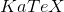is now capable of doing a bunch of environments like array and matrices. Aligning equation can get by with the aligned environments, lots of symbols are added as well.

That being said, it would be great to see the equation/align environment being supported. With the speed of, it can be a game-changers for general users. Not sure if it's suitable for power users of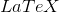who are more interested in using packages, referencing and other fancy features though. It's also unclear how the speed will be affected asexpands its library over time, but so far so good.

19. Sebastian says:

What You can say about "fast-preview" configuration option in MathJax is it effective?

20. Murray says:

@Sebastian: I see you are using fast preview on your site. On each page, I could see around 5 or 6 re-draws of the math until it finally settled down. KaTeX avoids all that which is why I use it almost exclusively now.

### Comment Preview

HTML: You can use simple tags like <b>, <a href="...">, etc.

To enter math, you can can either:

1. Use simple calculator-like input in the following format (surround your math in backticks, or qq on tablet or phone):
a^2 = sqrt(b^2 + c^2)
(See more on ASCIIMath syntax); or
2. Use simple LaTeX in the following format. Surround your math with $$ and $$.
$$\int g dx = \sqrt{\frac{a}{b}}$$
(This is standard simple LaTeX.)

NOTE: You can mix both types of math entry in your comment.